View on GitHub

## Interpolating Scattered Data in N-Dimensions

This project explores the use of Radial Basis Functions (RBFs) in the interpolation of scattered data in N-dimensions. It was completed Summer 2014 by Jesse Bettencourt as an NSERC-USRA student under the supervision of Dr. Kevlahan in the Department of Mathematics and Statistics at McMaster University, Hamilton, Ontario, Canada.

*Undergraduate Student Research Awards (USRA) are granted by the Natural Sciences and Engineering Research Council of Canada to 'stimulate interest in research in the natural sciences and engineering' and to encourage graduate studies and the pursuit of research careers in these fields.

This repository contains resources and working documents associated with the project. The early stages of the project focused on reviewing the published literature on RBF interpolation. This was summarized in a presentation given at the Canadian Undergraduate Mathematics Conference (CUMC) 2014 at Carelton University. The pdf, LaTeX, and figures as well as the python script to generate figures from this presentation can be found in the CUMC Presentation folder. Following the presentation, the project shifted focus to demonstrating the SciPy implementation of RBF interpolation. The Interpolation Demonstration folder contains the python files associated with this exploration. Of note, the file SphericalHarmonicInterpolation.py demonstrates how RBFs can be used to interpolate spherical harmonics given data sites and measurements on the surface of a sphere. This folder also contains iPython notebooks from early experimentation with SciPy's RBF and a Mathematica notebook from preliminary assessment of Mathematica implementation of RBF interpolation.

## What is interpolation?

Given a set of measurements $\{f_i\}_{i=1}^N$ taken at corresponding data sites $\{x_i\}_{i=1}^N$ we want to find an interpolation function $s(x)$ that informs us on our system at locations different from our data sites.A set of data sites $\{x_i\}_{i=1}^N$ each with an associated measurment $\{f_i\}_{i=1}^N$ interpolated using an interolation function $s(x).$

Further, we want our function, $s(x)$ to satisfy what's called the interpolation condition which is that we want to our interpolation function to exactly match our measurements at our data sites.

Interpolation Condition: \begin{equation} s(x_i)=f_i \end{equation} $\forall i\in{0 ... N }$

This is how interpolation differs from approximation, where approximation does not necessitate that our function exactly equals our measurements at the data sites. This can be achieved through different methods, e.g., Least Squares approximation. Sometimes, when accuracy at data sites is not necessary, approximation is preferred over interpolation because it can provide a 'nicer' function which could better illustrate the relationship among the data sites and measurements. For instance, approximation is heavily utilized in experimental science where measurements can contain a measurement error associated with experimental procedures. In this environment, the interpolation condition may be undesirable because it forces the interpolation to match exactly with potential measurement error, where approximation may alleviate error influence and illustrate measured correlations better.By the interpolation condition, an interpolation function must exactly match the measurments at our data sites. Approximation, on the other hand, does not have this requirment and may not move through our measurment sites.

For the purposes of this project, we focus on interpolation only.

## Interpolation Assumption

Many interpolation methods rely on the convenient assumption that our interpolation function, $s(x)$, can be found through a linear combination of basis functions, $\psi_i(x)$.

Linear Combination Assumption: \begin{equation*} s(x)=\sum_{i=1}^N \lambda_i \psi_i \end{equation*}

This assumption is convenient as it allows us to utilize solving methods for systems of linear equations from linear algebra to find our interpolation function. As such, we can express our interpolation problem as a linear system.

Interpolation as Linear System: \begin{equation*} A\boldsymbol{\lambda}=\boldsymbol{f} \end{equation*}

Where $\boldsymbol{f}$ is the vector of datasite measurements $\left[ f_1, ..., f_N \right]^T$ and $\boldsymbol{\lambda}$ is the vector of linear combination coefficients $\left[ \lambda_1, ..., \lambda_N \right]^T$.

For a system with N measurement data sites, $A$ is an NxN-matrix called the interpolation matrix or Vandermonde Matrix The elements of A are given by the basis functions, $\psi_j$ evaluated at each data site, $x_i$.

Elements of $A$: \begin{equation*} a_{ij}=\psi_j(x_i) \end{equation*}

By using numerical methods and solving this linear system, we will have our interpolation function as a linear combination of our basis functions.

#### Familiar Example of Interpolation Basis

A choice of basis functions, $\psi_i$, which may familiar to undergraduate students is the basis of (N-1)-degree polynomials. If we wish to find a 1-Dimensional interpolation function from N distinct data sites, we can find an (N-1)-degree polynomial which goes exactly through all sites. In other words, by choosing our basis functions to be successive powers of x up to (N-1), we can solve our interpolation system for our function.

Polynomial Interpolation Basis: \begin{equation*} \psi_{i=1}^N={1,x,x^2,x^3, ..., x^{N-1}} \end{equation*}

An example of this interpolation with 6 data sites can be seen in Figure 3. Here the interpolation function, as a linear combination, is $s(x)=-0.02988 x^5 + 0.417 x^4 - 2.018 x^3 + 3.694 x^2 - 1.722 x - 5.511e^{-14}$Interpolation of 6 data sites by linear combination. The basis functions are succesive powers of $x$ up to $x^5$.

However, while polynomial basis is simple for 1-Dimensional interpolation, this method is not ideal for higher dimensions. To accommodate higher dimension interpolation, we must choose our basis differently.

## Well-Posedness in Higher Dimensional Interpolation

When defining our linear system we must consider whether our system is well-posed. That is, does there exist a solution to our interpolation problem, and if so is that solution unique?

Well-Posedness in Linear Systems:

Our system will be well-posed if and only if $A$ is non-singular, i.e. $\det(A)\neq0$

For 1-D interpolation, many choices in basis functions will guarantee a well-posed system. In our example of polynomial interpolation, for instance, it was guaranteed that for N-distinct data sites a unique (N-1)-degree polynomial will interpolate the measurements. So without predetermining any information about our data sites (other than that they are distinct from each other), or their measurements, we can define our basis functions independently of our data and expect a unique, well-posed solution.

However, for n-Dimensions where $n\geq2$ this is never guaranteed! That is, no matter what we choose for our set of basis functions, there will always be data sites which produce ill-posed systems. The implication of this is that we can not define our basis functions independently of our data and expect a well-posed system. This results from the Haar-Mairhuber-Curtis Theorem.

### Haar-Mairhuber-Curtis Theorem

Through the work of Alfréd Haar and his description of Haar Spaces we gain the negative result that well-posedness is not guaranteed in higher dimensional linear systems with independently chosen basis functions. To state the theorem we first define Haar spaces.

Definition of Haar Space:

Let $\Omega \subset \mathbb{R}^N$ be a set with at least $N$ sites in it. Let $V \subset C(\Omega)$ be an $N$-dimensional subspace of continuous functions. Then, we say that $V$ is a Haar Space if for any collection of $N$ sites ${x_1,...,x_N}$ with any corresponding set of values ${f_1,...,f_N}$, we can find a unique function $s \in V$ such that $s(x_k)=f_k$.

From this definition we have the following lemma.

Lemma:

Let $\Omega \subset \mathbb{R}^N$ be a set with at least $N$ sites in it and $V \subset C(\Omega)$ be a subspace. Then, $V$ is an $N$-dimensional Haar Space if and only if for any distinct sites ${x_1,...,x_N} \in \Omega$ and any basis of functions ${\psi_1,...,\psi_N} \in V$, we have $\det(\psi_j(x_i))\neq0$.

In other words:

$V$ is a Haar Space $\iff$ any set of basis function produce well-posed system for any set of distinct data sites.

For the purposes of interpolation, then, interpolating within a Haar space is ideal, because then we can choose our basis independently of our data and, as per the lemma, we are guaranteed a well-posed system and a unique solution.

However, by the negative result of the Mairhuber-Curtis Theorem, there can be no Haar Spaces in $N$-Dimensions for $N \geq 2$

Mairhuber-Curtis Theorem:

Let $\Omega \subset \mathbb{R}^N$, $N \geq 2$ contain an interior site. Then, there is no Haar space of dimension $N \geq 2$ for $\Omega$.

So, if we are interpolating scattered data in higher dimensions, by the Haar-Mairhuber-Curtis Theorem we cannot choose our basis functions independent from our data sites. However, this does not mean we cannot interpolate in higher dimensions using our interpolation assumption.

If we can't guarantee well-posedness with independently chosen basis functions, we must choose our basis functions depending on our data sites.

## Basis Functions for Higher Dimension Interpolation

One method for defining basis functions depending on our data sites is to take a single function and translate it for each site. That is, our basis functions will be translates of a single function for each data site.

### Translates of the Basic Function

If our basis functions are translates of a function, which function should we translate? By answering this question we will arrive at the definition of Radial Basis Functions, but first let's consider a preliminary function: the basic function.

The Basic Function: \begin{equation*} \psi_i(x)=||x-x_i|| \end{equation*}

The basic function (Figure 5) is the absolute valued function given by the Euclidean distance from a center point $x_i \in \mathbb{R}^N$. The basic function has the feature that it is radially symmetric about this center point.The basic function is given by the Euclidean distance from a center, $x_i \in \mathbb{R}^N$. As such, the basic function is radially symetric about its center.

We can define our set of basis functions, $\{\psi_i(x)\}_{i=1}^N$, as translates of our basic function such that the center points are located at our data sites.

Set of Basis Functions: \begin{equation*} {\psi_i(x)=||x-x_i||}_{i=1}^N \end{equation*}

In other words, our set of basis functions is composed of basic functions centered each of our data sites. We can visualize one of these centered basic functions in Figure 6.In our set of basis functions $\{\psi_i(x)\}_{i=1}^N$, our $i^{th}$ function, $\psi_i(x)$, is a translation of the basic function so that it is centered on our $i^{th}$ data site, $x_i$.

Now that we have chosen our basis functions, we can look at the linear system which it produces. For instance, our interpolation matrix, $A$, now becomes: \begin{equation*} A= \begin{bmatrix} ||x_1-x_1|| & ||x_1-x_2|| & \cdots & ||x_1-x_N||\\ ||x_2-x_1|| & ||x_2-x_2||& \cdots & ||x_2-x_N||\\ \vdots & \vdots & \ddots & \vdots\\ ||x_N-x_1|| & ||x_N-x_2||& \cdots & ||x_N-x_N|| \end{bmatrix} \end{equation*}

This matrix is known as the distance matrix with Euclidean distance.

But, if we're not interpolating in 1-D, then we know we're not in a Haar Space. How do we know that our linear interpolation system with the distance matrix is well-posed?

Lemma from Linear Algebra: Distance Matricies with Euclidean distance, for distinct points in $\mathbb{R}^n$ are always non-singular.

From the above lemma we know that our interpolation matrix is non-singular. Therefore, we know our system is well-posed and that there exists a unique interpolation function!

However, the choice of $\psi_i(x)=||x-x_i||$ as our basic function is not ideal. As we can see from our above plot of the basic function centered at $x_i$, the first derivative of the basic function is discontinuous at our center point, $x_i$. This has the consequence that, at each of our data sites, the first derivative of our interpolation function will be discontinuous. This is problematic because, ideally, we would like to have a $C^\infty$ smooth interpolation function so we can use methods from calculus to analyze our function.

How can we remedy our derivative discontinuities in our interpolation function?

### Building a Better Basic Function

In 1968, R.L. Hardy suggested that by using a $C^\infty$ smooth function as our basic function, we can produce smooth interpolation functions. These functions are called Kernels. The kernel suggested by Hardy was the Multiquadric Kernel.

Hardy's Multiquadric Kernel: \begin{align*} \psi(x)=\sqrt{c^2 + x^2} \end{align*} where $c \neq 0$.

Notice that if we allow $c=0$ in the multiquadric kernel then we are actually describing the basic function used above. So, in other words, Hardy's multiquadric kernel is like the basic function but smoothed with a parameter $c$. By looking at a plot of the multiquadric kernel, we can see that the discontinuity from the basic function has been addressed. In fact, the multiquadric function is, as desired, $C^\infty$ smooth.Hardy's Multiquadric Kernel is $C^\infty$-smooth and shaped by a parameter $c$

As before, we will define our basis functions, ${\psi_i}_{i=1}^{N}$, as a set of multiquadric kernels translated such that they are centered at our data sites, $x_i$.

Basis of Multiquadric Kernels: \begin{equation*} {\psi_i(x)=\sqrt{c^2 + (||x-x_i||)^2}}_{i=1}^N \end{equation*}

We can visualize one of these translated multiquadric kernels in the figure below.In our set of basis functions $\{\psi_i(x)\}_{i=1}^N$, our $i^{th}$ function, $\psi_i(x)$, is a translation of Hardy's Multiquadric Kernel function so that it is centered on our $i^{th}$ data site, $x_i$.

Notice that the multiquadric kernel is also radially symmetric about its center, $x_i$. Because of this radial symmetry, the multiquadric kernel can be described as a Radial Basis Function. In other words, it is a basis function which depends only on the radial distance from its center. Since our basis functions $\psi_i(x)$ depend only on distance, we can re-express them as such.

Radial Basis Functions: \begin{equation*} \psi(||x-x_i||)= \phi(r) \end{equation*} where $r=||x-x_i||$

With our interpolation assumption, we can express our interpolation function as a linear combination of these functions, as before:

Interpolation as Linear Combination of Radial Basis Functions: \begin{equation*} s(x)=\sum_{i=1}^N \lambda_i \psi(||x-x_i||)=\sum_{i=1}^N \lambda_i \phi(r) \end{equation*}

There are a few commonly used radial basis function kernels:

• Multiquadric: $\phi(r)=\sqrt{1+(\epsilon r)^2}$• Inverse Multiquadric: $\phi(r)=\frac{1}{\sqrt{1+(\epsilon r)^2}}$• Inverse Quadratic: $\phi(r)=\frac{1}{1+(\epsilon r)^2}$• Gaussian: $\phi(r)=e^{-(\epsilon r)^2}$As before, we can use translates of these functions centered on our data sites as basis for our interpolation linear system. Further, notice that the multiquadric kernel has been rearranged to replace $c$ with a shape parameter, $\epsilon$ consistent with the other kernels.

However, by using the radial basis kernels as our basis, we change the interpolation matrix so that it is no longer the distance matrix as before.

Interpolation matrix with RBF kernels: \begin{equation*} A= \begin{bmatrix} \phi_1(r_1) & \phi_1(r_2) & \cdots & \phi_1(r_N)\\ \phi_2(r_1) & \phi_2(r_2)& \cdots & \phi_2(r_N)\\ \vdots & \vdots & \ddots & \vdots\\ \phi_N(r_1) & \phi_N(r_2)& \cdots & \phi_N(r_N) \end{bmatrix} \end{equation*}

If $N\geq2$ then we are still not interpolating in a Haar Space, and since we are no longer using a distance matrix, can we expect well-posedness?

To answer this question we determine if our interpolation matrix is positive-definite.

A matrix, $A$, is positive-definite if \begin{align*} & t^TAt>0 & \forall t=\left[ t_1, t_2, ..., t_n\right]\neq 0 \in \mathbb{R}^n \end{align*}

Using this definition we have the following condition:

If interpolation matrix, $A$, is symmetric, positive-definite , then $A$ is nonsingular and our system is well-posed.

So we can guarantee the existence of a unique solution if we choose our kernels such that $A$ will be positive-definite. In fact, we can produce positive-definite interpolation matrices by using positive-definite kernels.

A function, $\phi: \mathbb{R}^n\times \mathbb{R}^n \rightarrow \mathbb{R}$, is said to be positive definite if : \begin{align*} &\sum_{i=1}^N \sum_{j=1}^N \phi(||x-x_i||)t_i\bar{t_j}>0 &\forall t=\left[ t_1, t_2, ..., t_n\right]\neq 0 \in \mathbb{C}^n \end{align*}

Of the common RBF kernels described above, all are positive-definite except Hardy's Multiquadric kernel. However, the multiquadric kernel is guaranteed to produce well-posed systems for other, similar reasons (that it is conditionally negative-definite). With the exception of Hardy's multiquadric kernel, by using positive-definite kernels we can produce positive-definite interpolation matrices which guarantee well-posed systems!

So, by using Radial Basis Kernels for interpolation, we have shown that there exists a unique interpolation function $s(x)$ which interpolates scattered data in N-dimensions.

Radial Basis Interpolation \begin{equation*} s(x)=\sum_{i=1}^N \lambda_i \psi(||x-x_i||)=\sum_{i=1}^N \lambda_i \phi(r) \end{equation*}

### Well-posed v.s. Well-conditioned

In the discussion above we have shown that radial basis interpolation is well-posed, so there exists a unique solution for the interpolation problem. However, because these systems are solved using numerical methods on computers, they are subject to computational limitations. By using computational methods we introduce a complication, just because a solution exists, doesn't mean that it is accessible through numerical methods. A common example of the limitations that can cause a solution to be inaccessible is the accumulation of rounding errors. If our solution exists, and the system behaves 'nicely' with computational solving methods, then we say the solution is well-conditioned.

Radial basis interpolation problems, although well-posed, have the propensity to be very ill-conditioned. This is in part due the choice shape parameter, $\epsilon$. For some systems, small changes in $\epsilon$ may have potentially significant influences on the system.

In the two figures below we can see how increasing the value of epsilon will change the shape of the individual kernel basis functions.

For $\epsilon=0.4$For $\epsilon=1$In the three figures below, we can see how increasing the value of epsilon will cause the interpolation system to become ill-conditioned. Keep in mind that the interpolation solution for each $\epsilon$ value still exists, but the computation methods create noise and are unable to find the function.So we can see that in order to use radial basis function interpolation we must choose epsilon in such a way that the system does not become ill-conditioned.

Another limitation of radial basis function interpolation is that any error that occurs, as with ill-conditioning, occurs to a greater extent near the boundaries. This can be seen in the above three figures as the solution becomes more noisy, the noise is greater at the boundaries. This is because radial basis function interpolation relies on the radial symmetry of the basis functions. Basis functions centered at data sites on or close to the boundaries of the interpolation space become asymmetric. Of course, this can be avoided entirely by using radial basis function interpolation to interpolate functions in spaces without boundaries, e.g. surface of a sphere.

## Demonstrating Radial Basis Interpolation on Surface of Sphere

As part of this project I demonstrate how SciPy's implimentation of Radial Basis Function interpolation can be used to interpolate spherical harmonic functions on the surface of a sphere. The complete code for this demonstration can be found in this repository under the Interpolation Demonstration folder in the file SphericalHarmonicInterpolation.py.

### Dependencies

To fully use the python code you need the following libraries:

### Setting Up Coordinates

There are two sets of points used throughout the code. The data sites, which is used to train the RBF interpolation, called coarse coordinates, and the interpolation sites, where the function is being interpolated, called fine coordinates. I define the function which produces both set of points

def coordinates(n_fine, n_coarse):


where n_fine and n_coarse are parameters given to define the resolution of the interpolation space and the number of data sites respectively. Because of the way the interpolation space grid is defined, n_fine is given as a complex number.

#### Fine Coordinate Grid

The interpolation space grid, produced by the function make_coor(n) called with n_fine produces a grid of points on a sphere corresponding to latitude-longitude style grid points. In other words, the function creates an (n x n)-sized grid of points on the ($\phi, \theta$)-space. Then the function converts those points to Cartesian (x,y,z)-coordinates.

    def make_coor(n):
'''Creates points on the surface of sphere using lat-lon grid points'''
phi, theta = np.mgrid[0:pi:n, 0:2 * pi:n]
Coor = namedtuple('Coor', 'r phi theta x y z')
r = 1
x = r * sin(phi) * cos(theta)
y = r * sin(phi) * sin(theta)
z = r * cos(phi)
return Coor(r, phi, theta, x, y, z)


The resulting points and spherical mesh can be seen in the figure below for n_fine = 20j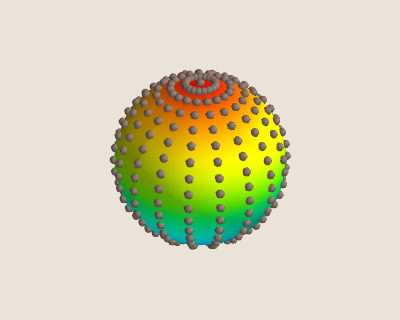From the figure we can see that the fine grid produces points at a much higher density at the poles than along the 'equator'. For this reason, we cannot use these points to train our radial basis function. Instead we must use a different method to produce our data sites.

#### Coarse Coordinate (Data Sites) Grid

For the RBF interpolation of an arbitrary function on the surface of a sphere we want to choose our points so they are equally spaced from each other. As it happens, the problem of uniformly distributing n-many points on the surface of a sphere is an open problem. For a large ($N \geq 10$) number of points, a sufficient method for pseudo-uniformly distributing $N$ points on the surface of the sphere is the Golden Section Spiral method. This algorithm places the points according to the golden spiral and can be visualized in the video below.

This algorithm was implemented in my code as the following function

    def uniform_spherical_distribution(N):
"""n points distributed evenly on the surface of a unit sphere"""
pts = []
r = 1
inc = pi * (3 - sqrt(5))
off = 2 / float(N)
for k in range(0, int(N)):
y = k * off - 1 + (off / 2)
r = sqrt(1 - y * y)
phi = k * inc
pts.append([cos(phi) * r, y, sin(phi) * r])
return np.array(pts)


However, since this only produces the Cartesian coordinates for these points and we will need the spherical coordinates, a we also append the spherical coordinates using the function:

    def appendSpherical_np(xyz):
'''Appends spherical coordinates to array of Cartesian coordinates'''
ptsnew = np.hstack((xyz, np.zeros(xyz.shape)))
xy = xyz[:, 0] ** 2 + xyz[:, 1] ** 2
ptsnew[:, 3] = np.sqrt(xy + xyz[:, 2] ** 2)
# for elevation angle defined from Z-axis down
ptsnew[:, 4] = np.arctan2(np.sqrt(xy), xyz[:, 2])
# ptsnew[:,4] = np.arctan2(xyz[:,2], np.sqrt(xy)) # for elevation angle
# defined from XY-plane up
ptsnew[:, 5] = np.arctan2(xyz[:, 1], xyz[:, 0])
return ptsnew


where parameter xyz is an array of Cartesian coordinates.

To produce our uniformly spaced coarse coordinates for interpolation, we call the function

    def make_uni_coor(n):
'''Make named tuple of unifromly distrubed points on sphere'''
Coor = namedtuple('Coor', 'theta phi x y z')
pts = uniform_spherical_distribution(n)
pts = appendSpherical_np(pts)

return Coor(pts[:, 5], pts[:, 4], pts[:, 0], pts[:, 1], pts[:, 2])


However, we've only generated the points of the sphere without providing information on how these points relate to each other. If we wish to plot these points as though they all belong on the surface of the sphere, we need to define a mesh. For this we use Delaunay triangulation to produce triangles between three adjacent points on the sphere.

To do this, we use DiPy's sphere object which allows us to define a sphere using our Cartesian coordinates. The object has the method faces() which are an array of triangles for our Delaunay mesh.

We can visualize our pseudo-uniformly distributed points and their Delaunay mesh in the figure below for n_coarse = 100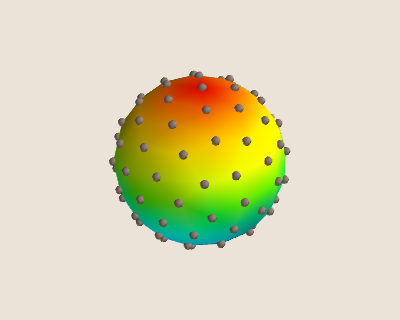### Using Named Tuples

The coordinate systems produced above use python's namedtuples as a variable naming convention. For instance, when defining our fine spherical grid we first define a namedtuple:

Coor = namedtuple('Coor', 'r phi theta x y z')


Then, when the function is returning the coordinates, it stores them as a named tuple as follows:

return Coor(r, phi, theta, x, y, z)


As such we can address the coordinates by selecting named elements of the tuple. The parent function for the producing the coordinates stores the fine and coarse coordinates as named tuples.

def coordinates(n_fine, n_coarse):
...
Coordinates = namedtuple('Coordinates', 'fine coarse ')

return Coordinates(make_coor(n_fine), make_uni_coor(n_coarse))


Now, if we generate our coordinates by calling this function and naming the output Coor.

Coor = coordinates(n_fine, n_coarse):


We can access all our coordinate information by addressing named elements inside the tuple. For instance, if we wish to address the fine coordinate's $\phi$ component, we can do so as follows Coor.fine.phi

Using named tuples for variable names allows for flexible and readable python code.

### Interpolating function

Now that we have our coordinates we can define a function on the surface of the sphere at those coordinates. For an example function on a sphere's surface we use the real part of spherical harmonics. Specifically, we use the real part of spherical harmonic $Y^3_4$.

To implement this, we use SciPy's Spherical Harmonic Function and define a function:

def harmonic(m, l, coor):
'''Produce m,l spherical harmonic at coarse and fine coordinates'''
Harmonic = namedtuple('Harmonic', 'fine coarse')
return Harmonic(
special.sph_harm(m, l, coor.fine.theta, coor.fine.phi).real,
special.sph_harm(m, l, coor.coarse.theta, coor.coarse.phi).real
)


Again, we make use of the namedtuple to define a fine and coarse harmonic. Since we are using RBF to interpolate the spherical harmonic from the coarse sites, we technically only need to evaluate the harmonic at the coarse coordinates. However, we are also interested in comparing the interpolated result to the actual function, to do this we also find the values of the function at the fine coordinates.

We call our function using our coordinates

function = harmonic(3, 4, coordinates)


### Implementing the Radial Basis Function Interpolation

We use SciPy implementation of RBF interpolation to define a function:

def rbf_interpolate(coor, coarse_function, epsilon=None):
'''Radial Basis Function Interpolation from coarse sites to fine cooridnates'''
# Train the interpolation using interp coordinates
rbf = Rbf(coor.coarse.x, coor.coarse.y,
coor.coarse.z, coarse_function, norm=angle, epsilon=epsilon)
# The result of the interpolation on fine coordinates
return rbf(coor.fine.x, coor.fine.y, coor.fine.z)


We call our RBF interpolation using our coarse Cartesian coordinates and the value of the harmonic at those coordinates:

    rbf = Rbf(coor.coarse.x, coor.coarse.y,
coor.coarse.z, coarse_function, norm=angle, epsilon=epsilon)


Notice that can we provide a value for epsilon (if given None SciPy will compute a default value). Further, note that we define a norm. This is the distance metric used to determine the radial distance from the data sites. As a default, SciPy will use the Euclidean Distance as the distance norm. However, since we are training our function using Cartesian coordinates on the surface of the unit sphere, we must use a distance metric for points on the surface of that sphere.

For the $S^2$ distance norm we define a function to be called from our Rbf:

def angle(x1, x2):
'''Distance metric on the surface of the unit sphere'''
xx = np.arccos((x1 * x2).sum(axis=0))
xx[np.isnan(xx)] = 0
return xx


Now once we train our radial basis function, rbf() we can use it to interpolate the spherical harmonic on our fine coordinates:

return rbf(coor.fine.x, coor.fine.y, coor.fine.z)


### Optimizing our Choice of Epsilon

We can define errors of our interpolation to be the difference between the interpolated function and the actual spherical harmonic function at each of the fine coordinates. We define a python function to give us these values:

def interp_error(fine_function, interp_results):
'''Error between interpolated function and actual function'''
Error = namedtuple('Error', 'errors max')
errors = fine_function - interp_results
error_max = np.max(np.abs(errors))
return Error(errors, error_max)


Further, we can assess the overall error of the interpolation by using the maximum difference between the interpolation and the function and calling this value error_max.

If we preform multiple RBF interpolation, each with different values of the shape parameter, $\epsilon$, we can see how epsilon effects the maximum error of the interpolation. Further, we can use this to choose the epsilon which minimizes this error.

I plot the maximum error for increasing values of epsilon, colouring the optimal choice red.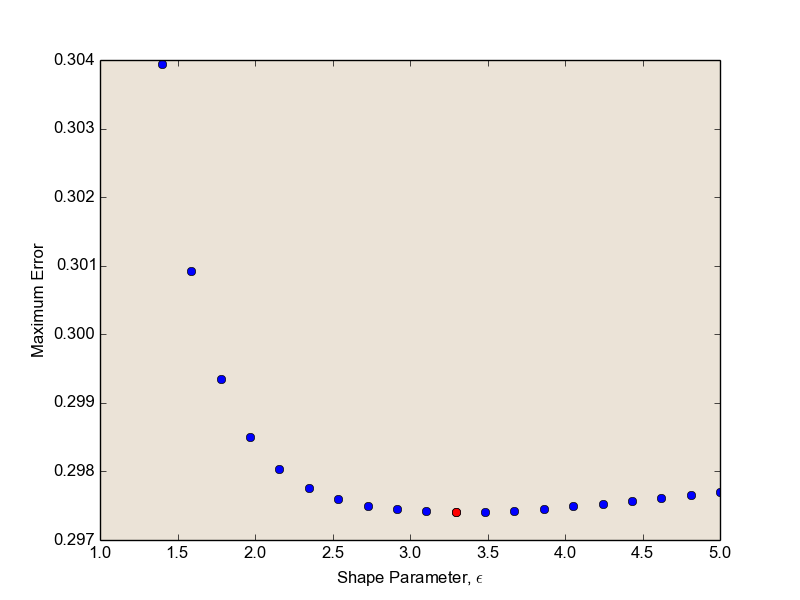By RBF interpolating our function with the optimal value of epsilon, we can minimize the interpolation error.

### Visualizing the Results of the Interpolation

Using the MayaVi scientific data visualization library we can visualize the results of this interpolation.

Note: The following images are stills from the MayaVi visualization environment, which is interactive. I highly recommend downloading and playing with these figures yourself, as you can rotate around the sphere.

First, we plot the spherical harmonic function on the sphere. We also add small 'warts' which indicate where the data sites being used for interpolation are, coloured to the value of the function at those sites.

 mlab.figure()
vmax, vmin = np.max(fun.fine), np.min(fun.fine)
mlab.mesh(coor.fine.x, coor.fine.y, coor.fine.z,
scalars=fun.fine, vmax=vmax, vmin=vmin)
mlab.points3d(coor.coarse.x, coor.coarse.y, coor.coarse.z, fun.coarse,
scale_factor=0.1, scale_mode='none', vmax=vmax, vmin=vmin)
mlab.colorbar(title='Spherical Harmonic', orientation='vertical')
mlab.savefig('Figures/functionsphere.png')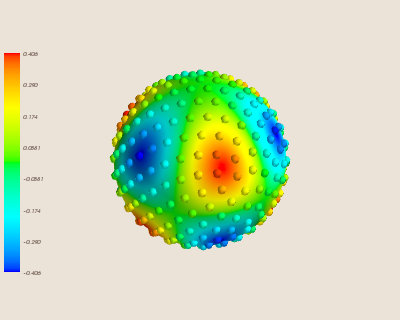Then, we can see the interpolated function: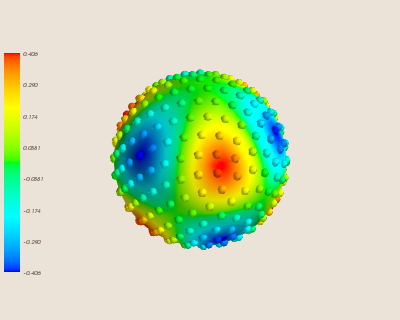Finally, we can see where the error occurs on our sphere by visualizing the error: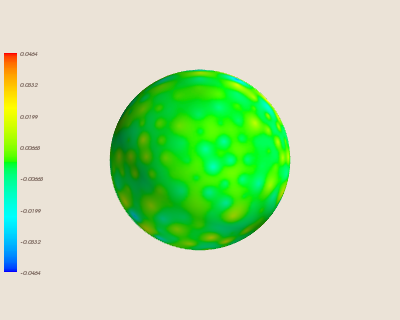Note that the above interpolation uses a relatively high number of data sites (N=350). We can see how this interpolation worsens with a fewer number of sites (N=100).

Again, here is the spherical harmonic we are interpolating with the 100 data sites.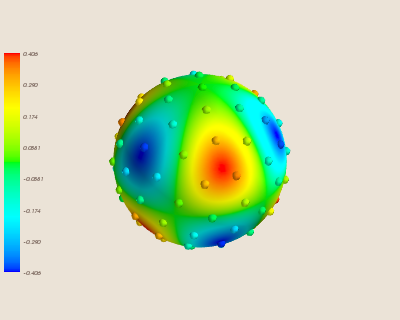Here is the interpolation trained with fewer sites.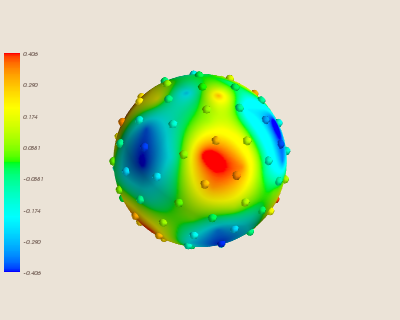Predictably, this causes the error of the interpolation to increase.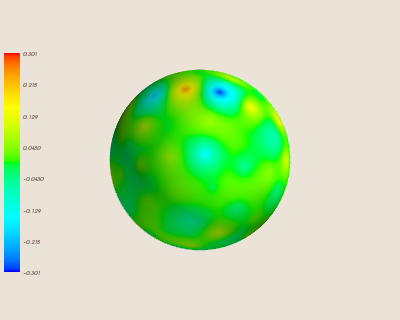### Conclusion

Radial Basis Interpolation is an effective method to interpolate high dimensional scattered data, especially if the interpolation space has no boundaries. The method solves the problem of Well-Posedness in higher dimensional scattered data, but still has the propensity to be ill-conditioned. However, like other interpolation methods, there are adjustments to RBF methods not discsussed in this project that can be used to improve the conditioning of the interpolation problem.

This project represented my introduction to interpolation theory, and as a summer project it was very rewarding to explore both theoretical aspect of Radial Basis Function interpolation while also applying the theory using computational methods. The project allowed me to develop knowledge of the Python language, including the implementation of SciPy and other common libraries. I also found great support from the Python community, especially through StackExchange, which supported a secondary goal of this project in exploring open-source community resources. This exploration also caused me to learn Git version control, and to keep my entire project stored on this GitHub, which was invaluable in managing my progress in the project, but also allowed me to easily communicate code and troubleshoot problems with peers and my supervisor. GitHub proved to be an excellent resource for this project, and allowed me to easily generate a website detailing my project through GitHub Pages, which links to the original repository and is easily accessible and digestible overview of my project. Through this project I was also given the oppurunity to present my research at the Canadian Undergraduate Mathematics Conference 2014 in Ottawa. This experience was extremely rewarding, and developed skills in presenting mathematical information that I had previously not explored.

Overall, the project was very successful in providing insight into mathematics research, and in developing my skills as a mathematician. I also gained many experiences outside of the mathematical focus, including Python literacy, version control, presentation methods, and access to the online support community. This project was extremely important to my undergraduate education, so thank you to NSERC for funding the USRA and to Dr. Nicholas Kevlahan for his supervision and guidance in the project.